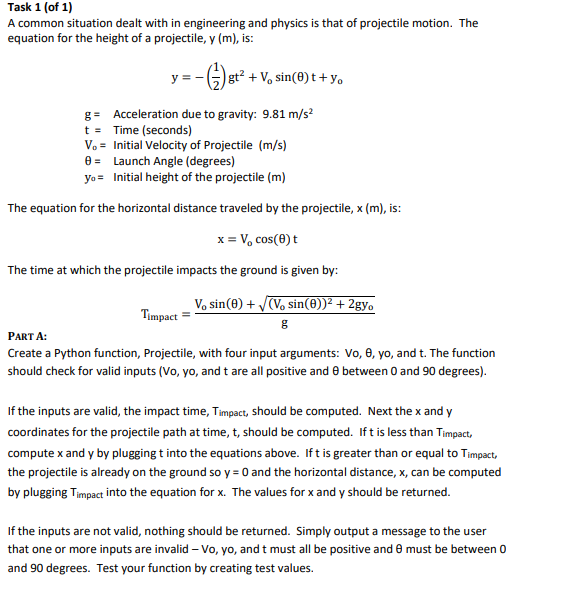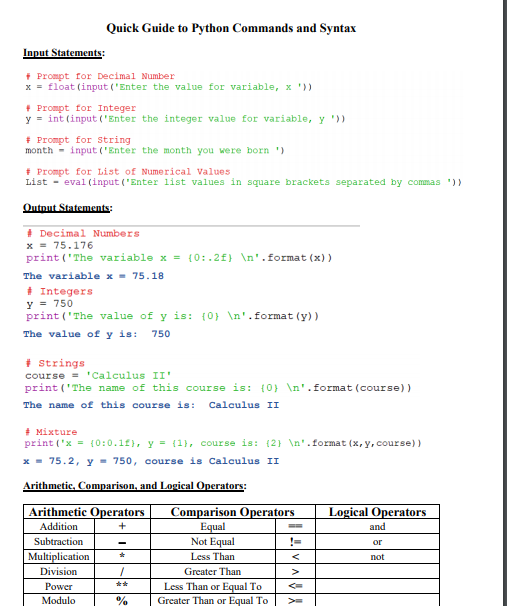# (Solved) : Task 1 1 Common Situation Dealt Engineering Physics Projectile Motion Equation Height Proj Q42751274 . . .Task 1 (of 1) A common situation dealt with in engineering and physics is that of projectile motion. The equation for the height of a projectile, y (m), is: y=-(,)st? + V, sin(e)t+yo g= Acceleration due to gravity: 9.81 m/s2 t = Time (seconds) Vo = Initial Velocity of Projectile (m/s) = Launch Angle (degrees) yo= Initial height of the projectile (m) The equation for the horizontal distance traveled by the projectile, x (m), is: x = V. cost The time at which the projectile impacts the ground is given by: V, sin(e) +(V, sin(())2 + 2gy. Timpact = PART A: Create a Python function, Projectile, with four input arguments: Vo, e, yo, and t. The function should check for valid inputs (vo, yo, and t are all positive and between 0 and 90 degrees). If the inputs are valid, the impact time, Timpact, should be computed. Next the x and y coordinates for the projectile path at time, t, should be computed. Ift is less than Timpact compute x and y by pluggingt into the equations above. Ift is greater than or equal to Timpact the projectile is already on the ground so y = 0 and the horizontal distance, x, can be computed by plugging Timpact into the equation for x. The values for x and y should be returned. If the inputs are not valid, nothing should be returned. Simply output a message to the user that one or more inputs are invalid – Vo, yo, and t must all be positive and must be between 0 and 90 degrees. Test your function by creating test values. Quick Guide to Python Commands and Syntax Input Statements + Prompt for Decimal Number x = float (input(‘Enter the value for variable, x ‘>) + Prompt for Integer y = int(input(‘Enter the integer value for variable, y » #Prompt for String month – input (“Enter the month you were born”) #Prompt for List of Numerical Values List – eval(input(‘Enter list values in square brackets separated by commas’)) Outnut Statements: Decimal Numbers X = 75.176 print(‘The variable x = {0:2} n’.format(x)) The variable x – 75.18 . Integers y = 750 print(‘The value of y is: (0) In’. format (y)) The value of y is: 750 Strings course = ‘Calculus II print(‘The name of this course is: {0} n’. format (course)) The name of this course is: Calculus II Mixture print(‘x = 10:0.17), y = {1}, course is: 12} n’. format (x, y, course) x – 75.2, Y-750, course is Calculus II Arithmetic. Comparison, and Logical Operators: Arithmetic Operators Addition + Subtraction – Multiplication Division Power ** Modulo 1 % Comparison Operators Equal Not Equal Less Than < Greater Than > Less Than or Equal To Greater Than or Equal Tol > Logical Operators and or not Show transcribed image text Task 1 (of 1) A common situation dealt with in engineering and physics is that of projectile motion. The equation for the height of a projectile, y (m), is: y=-(,)st? + V, sin(e)t+yo g= Acceleration due to gravity: 9.81 m/s2 t = Time (seconds) Vo = Initial Velocity of Projectile (m/s) = Launch Angle (degrees) yo= Initial height of the projectile (m) The equation for the horizontal distance traveled by the projectile, x (m), is: x = V. cost The time at which the projectile impacts the ground is given by: V, sin(e) +(V, sin(())2 + 2gy. Timpact = PART A: Create a Python function, Projectile, with four input arguments: Vo, e, yo, and t. The function should check for valid inputs (vo, yo, and t are all positive and between 0 and 90 degrees). If the inputs are valid, the impact time, Timpact, should be computed. Next the x and y coordinates for the projectile path at time, t, should be computed. Ift is less than Timpact compute x and y by pluggingt into the equations above. Ift is greater than or equal to Timpact the projectile is already on the ground so y = 0 and the horizontal distance, x, can be computed by plugging Timpact into the equation for x. The values for x and y should be returned. If the inputs are not valid, nothing should be returned. Simply output a message to the user that one or more inputs are invalid – Vo, yo, and t must all be positive and must be between 0 and 90 degrees. Test your function by creating test values.
Quick Guide to Python Commands and Syntax Input Statements + Prompt for Decimal Number x = float (input(‘Enter the value for variable, x ‘>) + Prompt for Integer y = int(input(‘Enter the integer value for variable, y » #Prompt for String month – input (“Enter the month you were born”) #Prompt for List of Numerical Values List – eval(input(‘Enter list values in square brackets separated by commas’)) Outnut Statements: Decimal Numbers X = 75.176 print(‘The variable x = {0:2} n’.format(x)) The variable x – 75.18 . Integers y = 750 print(‘The value of y is: (0) In’. format (y)) The value of y is: 750 Strings course = ‘Calculus II print(‘The name of this course is: {0} n’. format (course)) The name of this course is: Calculus II Mixture print(‘x = 10:0.17), y = {1}, course is: 12} n’. format (x, y, course) x – 75.2, Y-750, course is Calculus II Arithmetic. Comparison, and Logical Operators: Arithmetic Operators Addition + Subtraction – Multiplication Division Power ** Modulo 1 % Comparison Operators Equal Not Equal Less Than Less Than or Equal To Greater Than or Equal Tol > Logical Operators and or not

Answer to Task 1 (of 1) A common situation dealt with in engineering and physics is that of projectile motion. The equation for th…

We are the best freelance writing portal. Looking for online writing, editing or proofreading jobs? We have plenty of writing assignments to handle.